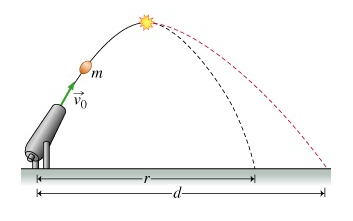# Problem: A mortar fires a shell of mass m at speed v0. The shell explodes at the top of its trajectory (shown by a star in (Figure 1) ) as designed. However, rather than creating a shower of colored flares, it breaks into just two pieces, a smaller piece of mass 1/5m and a larger piece of mass 4/5m. Both pieces land at exactly the same time. The smaller piece lands perilously close to the mortar (at a distance of zero from the mortar). The larger piece lands a distance d from the mortar. If there had been no explosion, the shell would have landed a distance r from the mortar. Assume that air resistance and the mass of the shell's explosive charge are negligible.Find the distance d from the mortar at which the larger piece of the shell lands

###### FREE Expert Solution

Law of conservation of momentum:

$\overline{){\mathbf{\sum }}{{\mathbf{p}}}_{{\mathbf{i}}}{\mathbf{=}}{\mathbf{\sum }}{{\mathbf{p}}}_{{\mathbf{f}}}}$

Since the shell was at the peak of its original trajectory when it exploded, its motion was purely horizontal: v = v0x

vx = v0cosθ

Using speed, distance and time relationship, v = d/t:

vx = (1/2r)/t = r/2t

Before the explosion, the momentum of the shell is:

p = mvx = mr/2t

The explosion occurred at a distance +(1/2)r from the mortar. The small piece covered a distance -(1/2)r towards the mortar. The negative sign shows direction.

The momentum of the smaller mass immediately after the explosion is given by:

83% (74 ratings)###### Problem Details

A mortar fires a shell of mass m at speed v0. The shell explodes at the top of its trajectory (shown by a star in (Figure 1) ) as designed. However, rather than creating a shower of colored flares, it breaks into just two pieces, a smaller piece of mass 1/5m and a larger piece of mass 4/5m. Both pieces land at exactly the same time. The smaller piece lands perilously close to the mortar (at a distance of zero from the mortar). The larger piece lands a distance d from the mortar. If there had been no explosion, the shell would have landed a distance r from the mortar. Assume that air resistance and the mass of the shell's explosive charge are negligible.Find the distance d from the mortar at which the larger piece of the shell lands